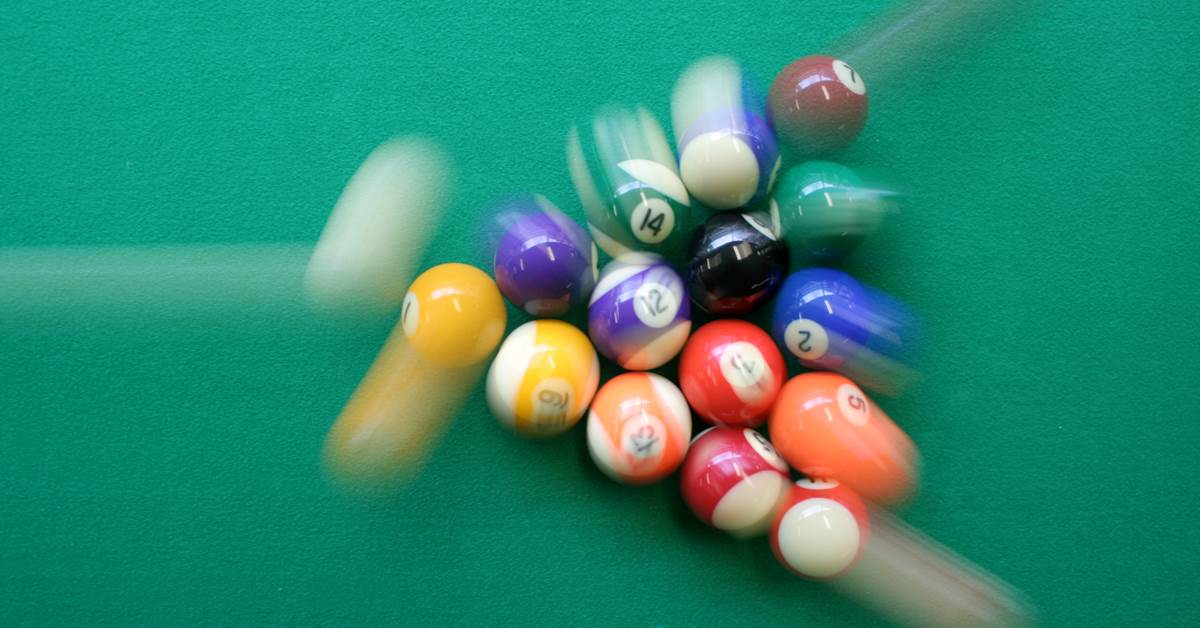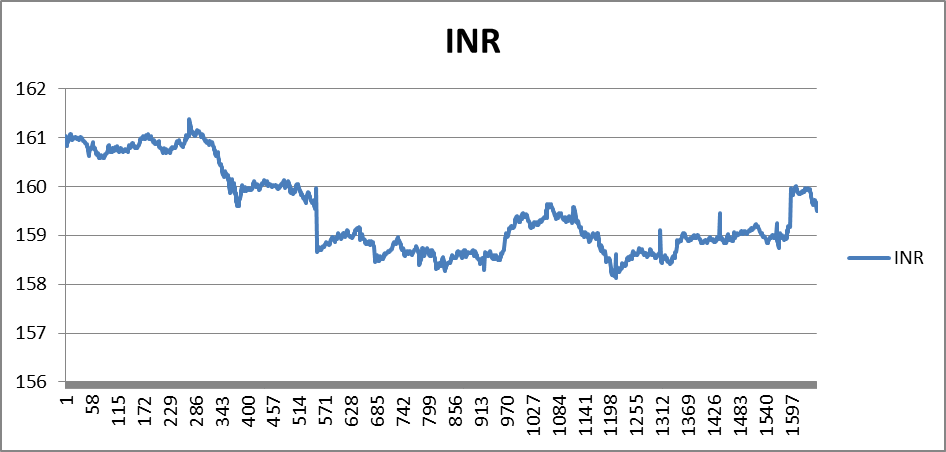# Candlestick Trading - A Momentum Strategy with ExampleCandle stick trading is a momentum strategy where you observe price on the previous ‘n’ candlesticks and make your bets accordingly. The intuition is if the price is increasing continuously for, say, 3 candle sticks then it is highly probable that it will rise further.

### How it helps?

• Learn how momentum strategy is implemented
• Understand the trading logic of strategy implementation

In this example we consider the INR FUTURES data on SGX (Singapore exchange). We implement momentum strategy on this contract. This momentum strategy is simply based on the fact that a rising market will be followed by a rising market and a falling market will be followed by a falling market. We hope to ride on the tide and make some profit before the momentum vanishes. The data used for INR FUTURES contract is the data separated by 5 minute interval for 3rd Feb 2015 to 19th March 2015. The trading strategy is intuitional and unlike mean reversion for pairs trading there is no hypothesis as such explaining why this strategy would work or not. We would like to benefit from the market wave and optimize our bet by specifying stop loss and take profit limits. This model is flexible and can be varied to achieve different limits to exit the trade depending upon the trader’s risk appetite.#### Assumptions

2. Prices are available at 5 minutes interval and we trade at the 5 minute closing price only.
3. Since this is discrete data, squaring off of the position happens at the end of the candle i.e. at the price available at the end of 5 minutes.
4. Only the regular session (T) is traded
5. Transaction cost is $0.35 for INR FUTURE. 6. Margin for each trade is$800.
7. Trading quantity is 1 lot and trading hours are 7:40 a.m. to 2:00 a.m. SGT.

#### Input parameters

Please note that all the values for the input parameters mentioned below are configurable.

• High/Low of 3 candles (one candle=every 5 minute price) is considered.
• A stop loss of 0.08 and profit limit of 0.16 is set.
• The order size for trading INR FUTURE is INR 2000000.

The market data and trading model are included in the spread sheet from the 12th row onwards. So when the reference is made to column D, it should be obvious that the reference commences from D12 onwards.

Column C represents the price for INR FUTURE.

Column D represents 3 candle high meaning the highest price of the previous 3 candles.

Column E represents 3 candle low meaning the lowest price of the previous 3 candles.

Column F calculates the trading signal. The formula =IF(D13="", "", IF(C13>D13, "Buy", IF(C13<E13, "Sell", ""))) means if the entry in cell D13 is blank then keep F13 blank otherwise if C13 (INR FUTURES data) is greater than D13 (3 candle high) then buy signal for the INR FUTURES contract is generated else if C13 is lower than E13 (3 candle low) then sell signal for the INR FUTURES contract is generated.

Column G represents entry price. This is the price at which the trading signal is generated. The formula =IF(H13=H12, G12, IF(OR(H13="Buy", H13="Sell"), C13, "")) means if the entry in cell H13 is same as H12 then the value in G13 should be the value in G12 otherwise if H13 is either “Buy” or “Sell” then the entry in G13 is the value in C13 (INR FUTURES price) else if H13 is neither “Buy” nor “Sell” leave it blank.

Column H represents the status of the trade. Given our assumptions and input parameters there are four status that can occur, “Buy”, “Sell”, “TP (Take Profit)” and “SL (Stop Loss)”.

The formula =IF(OR(H17="", H17="TP", H17="SL"), F18, IF(H17="Buy", IF(C18<G17+$C$4, "SL", IF(C18>G17+$C$5, "TP", H17)), IF(H17="Sell", IF(C18>G17-$C$4, "SL", IF(C18<G17-$C$5, "TP", H17)), ""))) can be simplified as follows:-

If the entry in H17 is either blank or TP or SL then choose the value in F18 (F column has either Buy or Sell or blank values). Otherwise look into the next If condition.

If the entry in H17 is “Buy”, meaning we have a buy position, and if the price of the contract goes below the stop loss limit then we exit the position at stop loss and if the price of the contract goes above the take profit limit then we exit the position at take profit. Similarly, if the position is “Sell” and the contract price rises above the selling price beyond the stop loss limit then exit the position at stop loss and if the contract price falls below the selling price beyond the take profit limit then exit the position by taking the profit.

Column I represents the profit/loss status of the trade. P/L is calculated only when we have squared off our position. The formula =IF(OR(H13="SL", H13="TP"), IF(H12="Buy", C13-G12, IF(H12="Sell", G12-C13, 0)), 0) can be summarized as follows:-

The first if condition states that proceed to the next if condition only if the corresponding status in column H is either “SL” or “TP” else the entry in the cell is zero.

The next set of if conditions calculate profit assuming either stop loss or take profit has been achieved. If the status in column H is “Buy”, then the profit/loss is calculated as C13-G12. Remember that the column G has the price at which you traded (in this case “Buy”) and the column C has the market data for INR FUTURES contract. Hence the profit/loss is simply the difference between the price at which you sold minus the price at which you bought. If the status in column H is “Sell”, then the profit/loss is calculated as G12-C13 simply meaning the difference between the price at which you sold ( shorted) and the price at which you bought later thus squaring off the position.

Column J calculates the cumulative profit.

#### Outputs

The output table has some performance metrics tabulated. Loss from all loss making trades is $1588 and profit from trades that hit TP is$1988. So the total P/L is $1988-$1588=$400. Loss trades are the trades that resulted in losing money on the trading positions. Profitable trades are the successful trades ending in gaining cause. Average profit is the ratio of total profit to the total number of trades. Net average profit is calculated after subtracting the transaction costs which amounts to$3.03.